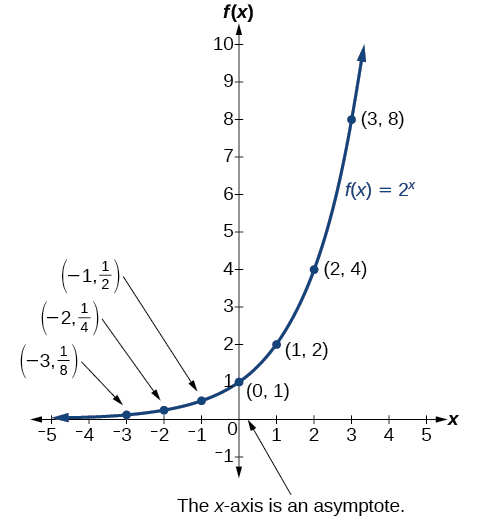# 6.2 Graphs of exponential functions

 Page 1 / 6
• Graph exponential functions.
• Graph exponential functions using transformations.

As we discussed in the previous section, exponential functions are used for many real-world applications such as finance, forensics, computer science, and most of the life sciences. Working with an equation that describes a real-world situation gives us a method for making predictions. Most of the time, however, the equation itself is not enough. We learn a lot about things by seeing their pictorial representations, and that is exactly why graphing exponential equations is a powerful tool. It gives us another layer of insight for predicting future events.

## Graphing exponential functions

Before we begin graphing, it is helpful to review the behavior of exponential growth. Recall the table of values for a function of the form $\text{\hspace{0.17em}}f\left(x\right)={b}^{x}\text{\hspace{0.17em}}$ whose base is greater than one. We’ll use the function $\text{\hspace{0.17em}}f\left(x\right)={2}^{x}.\text{\hspace{0.17em}}$ Observe how the output values in [link] change as the input increases by $\text{\hspace{0.17em}}1.$

 $x$ $-3$ $-2$ $-1$ $0$ $1$ $2$ $3$ $f\left(x\right)={2}^{x}$ $\frac{1}{8}$ $\frac{1}{4}$ $\frac{1}{2}$ $1$ $2$ $4$ $8$

Each output value is the product of the previous output and the base, $\text{\hspace{0.17em}}2.\text{\hspace{0.17em}}$ We call the base $\text{\hspace{0.17em}}2\text{\hspace{0.17em}}$ the constant ratio . In fact, for any exponential function with the form $\text{\hspace{0.17em}}f\left(x\right)=a{b}^{x},$ $\text{\hspace{0.17em}}b\text{\hspace{0.17em}}$ is the constant ratio of the function. This means that as the input increases by 1, the output value will be the product of the base and the previous output, regardless of the value of $\text{\hspace{0.17em}}a.$

Notice from the table that

• the output values are positive for all values of $x;$
• as $\text{\hspace{0.17em}}x\text{\hspace{0.17em}}$ increases, the output values increase without bound; and
• as $\text{\hspace{0.17em}}x\text{\hspace{0.17em}}$ decreases, the output values grow smaller, approaching zero.

[link] shows the exponential growth function $\text{\hspace{0.17em}}f\left(x\right)={2}^{x}.$Notice that the graph gets close to the x -axis, but never touches it.

The domain of $\text{\hspace{0.17em}}f\left(x\right)={2}^{x}\text{\hspace{0.17em}}$ is all real numbers, the range is $\text{\hspace{0.17em}}\left(0,\infty \right),$ and the horizontal asymptote is $\text{\hspace{0.17em}}y=0.$

To get a sense of the behavior of exponential decay , we can create a table of values for a function of the form $\text{\hspace{0.17em}}f\left(x\right)={b}^{x}\text{\hspace{0.17em}}$ whose base is between zero and one. We’ll use the function $\text{\hspace{0.17em}}g\left(x\right)={\left(\frac{1}{2}\right)}^{x}.\text{\hspace{0.17em}}$ Observe how the output values in [link] change as the input increases by $\text{\hspace{0.17em}}1.$

 $x$ $-3$ $-2$ $-1$ $0$ $1$ $2$ $3$ $g\left(x\right)=\left(\frac{1}{2}{\right)}^{x}$ $8$ $4$ $2$ $1$ $\frac{1}{2}$ $\frac{1}{4}$ $\frac{1}{8}$

Again, because the input is increasing by 1, each output value is the product of the previous output and the base, or constant ratio $\text{\hspace{0.17em}}\frac{1}{2}.$

Notice from the table that

• the output values are positive for all values of $\text{\hspace{0.17em}}x;$
• as $\text{\hspace{0.17em}}x\text{\hspace{0.17em}}$ increases, the output values grow smaller, approaching zero; and
• as $\text{\hspace{0.17em}}x\text{\hspace{0.17em}}$ decreases, the output values grow without bound.

[link] shows the exponential decay function, $\text{\hspace{0.17em}}g\left(x\right)={\left(\frac{1}{2}\right)}^{x}.$

The domain of $\text{\hspace{0.17em}}g\left(x\right)={\left(\frac{1}{2}\right)}^{x}\text{\hspace{0.17em}}$ is all real numbers, the range is $\text{\hspace{0.17em}}\left(0,\infty \right),$ and the horizontal asymptote is $\text{\hspace{0.17em}}y=0.$

## Characteristics of the graph of the parent function f ( x ) = b x

An exponential function with the form $\text{\hspace{0.17em}}f\left(x\right)={b}^{x},$ $\text{\hspace{0.17em}}b>0,$ $\text{\hspace{0.17em}}b\ne 1,$ has these characteristics:

• one-to-one function
• horizontal asymptote: $\text{\hspace{0.17em}}y=0$
• domain:
• range: $\text{\hspace{0.17em}}\left(0,\infty \right)$
• x- intercept: none
• y- intercept: $\text{\hspace{0.17em}}\left(0,1\right)\text{\hspace{0.17em}}$
• increasing if $\text{\hspace{0.17em}}b>1$
• decreasing if $\text{\hspace{0.17em}}b<1$

[link] compares the graphs of exponential growth    and decay functions.

Given an exponential function of the form $\text{\hspace{0.17em}}f\left(x\right)={b}^{x},$ graph the function.

1. Create a table of points.
2. Plot at least $\text{\hspace{0.17em}}3\text{\hspace{0.17em}}$ point from the table, including the y -intercept $\text{\hspace{0.17em}}\left(0,1\right).$
3. Draw a smooth curve through the points.
4. State the domain, $\text{\hspace{0.17em}}\left(-\infty ,\infty \right),$ the range, $\text{\hspace{0.17em}}\left(0,\infty \right),$ and the horizontal asymptote, $\text{\hspace{0.17em}}y=0.$

#### Questions & Answers

x exposant 4 + 4 x exposant 3 + 8 exposant 2 + 4 x + 1 = 0
x exposent4+4x exposent3+8x exposent2+4x+1=0
HERVE
How can I solve for a domain and a codomains in a given function?
ranges
EDWIN
Thank you I mean range sir.
Oliver
proof for set theory
don't you know?
Inkoom
find to nearest one decimal place of centimeter the length of an arc of circle of radius length 12.5cm and subtending of centeral angle 1.6rad
factoring polynomial
find general solution of the Tanx=-1/root3,secx=2/root3
find general solution of the following equation
Nani
the value of 2 sin square 60 Cos 60
0.75
Lynne
0.75
Inkoom
when can I use sin, cos tan in a giving question
depending on the question
Nicholas
I am a carpenter and I have to cut and assemble a conventional roof line for a new home. The dimensions are: width 30'6" length 40'6". I want a 6 and 12 pitch. The roof is a full hip construction. Give me the L,W and height of rafters for the hip, hip jacks also the length of common jacks.
John
I want to learn the calculations
where can I get indices
I need matrices
Nasasira
hi
Raihany
Hi
Solomon
need help
Raihany
maybe provide us videos
Nasasira
Raihany
Hello
Cromwell
a
Amie
What do you mean by a
Cromwell
nothing. I accidentally press it
Amie
you guys know any app with matrices?
Khay
Ok
Cromwell
Solve the x? x=18+(24-3)=72
x-39=72 x=111
Suraj
Solve the formula for the indicated variable P=b+4a+2c, for b
Need help with this question please
b=-4ac-2c+P
Denisse
b=p-4a-2c
Suddhen
b= p - 4a - 2c
Snr
p=2(2a+C)+b
Suraj
b=p-2(2a+c)
Tapiwa
P=4a+b+2C
COLEMAN
b=P-4a-2c
COLEMAN
like Deadra, show me the step by step order of operation to alive for b
John
A laser rangefinder is locked on a comet approaching Earth. The distance g(x), in kilometers, of the comet after x days, for x in the interval 0 to 30 days, is given by g(x)=250,000csc(π30x). Graph g(x) on the interval [0, 35]. Evaluate g(5)  and interpret the information. What is the minimum distance between the comet and Earth? When does this occur? To which constant in the equation does this correspond? Find and discuss the meaning of any vertical asymptotes.
The sequence is {1,-1,1-1.....} hasByByByBy David Martin